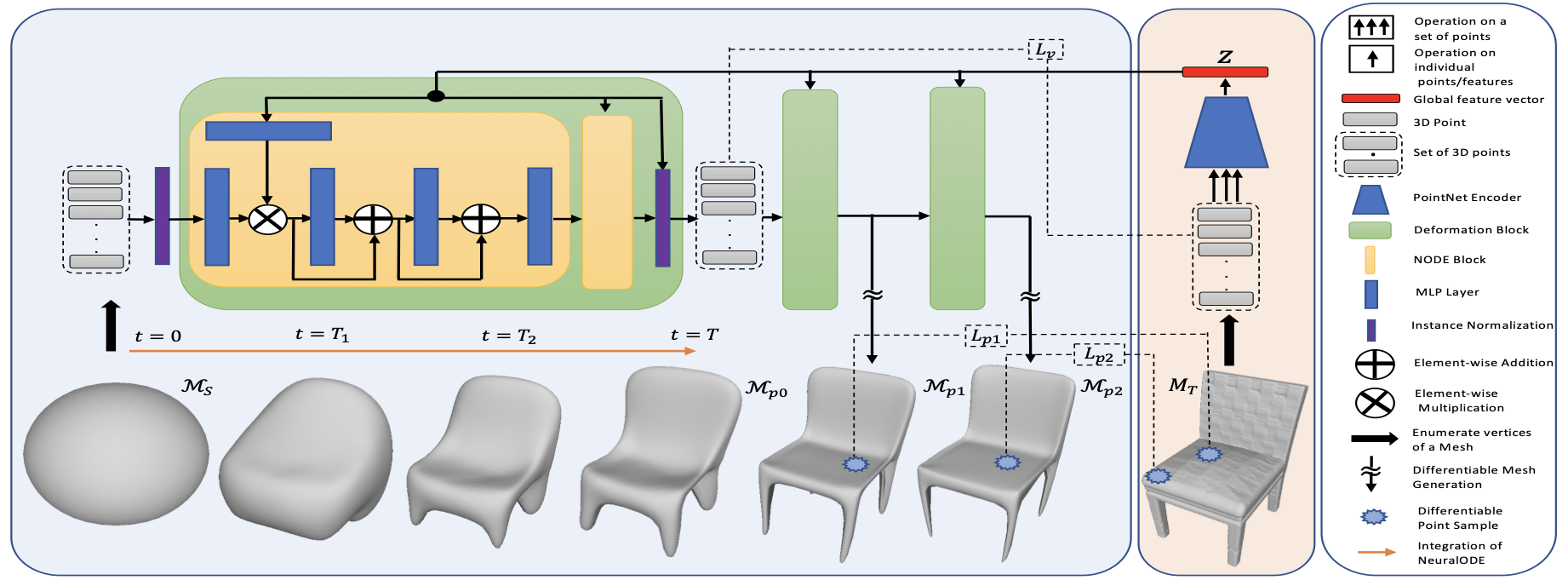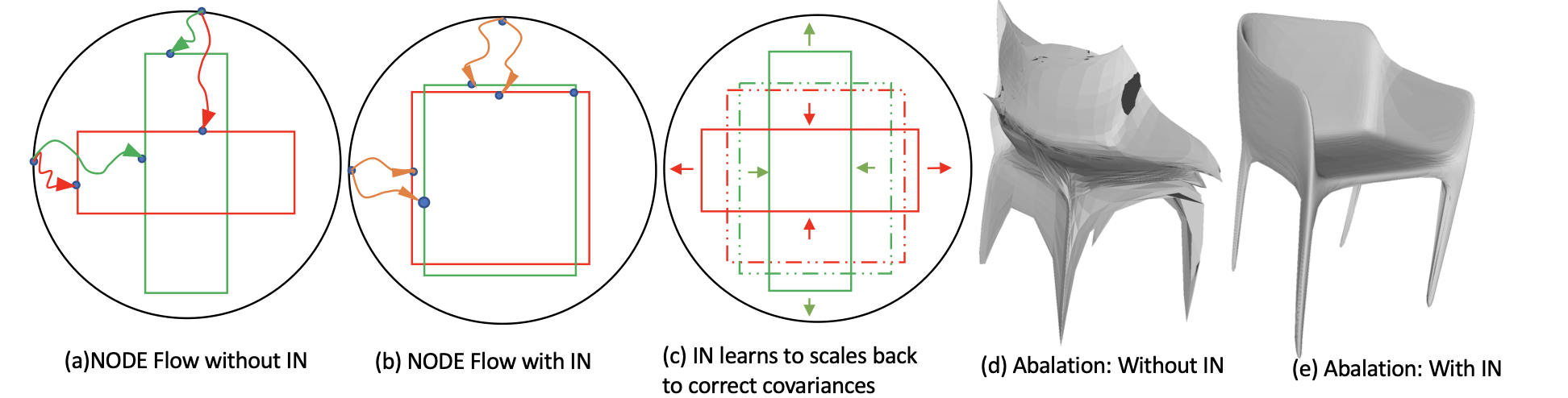# Creating 3D Meshes with Neural ODEs

Diffeomorphic Genus-0 Mesh Generation using Neural ODEs. Made by Adrish Dey using Weights & Biases

## Introduction

3D Meshes are the primary form of representing 3D objects computationally. They've found extensive usage in various arenas of science and technology, like virtual reality, physics simulations, game development, and manufacturing, to name just a few domains. But despite their extensive usage, capturing "directly usable" 3D objects is exceedingly difficult.
The primary problem that hinders the direct "plug-and-play" use of 3D models captured in the wild is the lack of "manifoldness," a property which describes if the represented 3D object will behave like a real-world object in a simulation, or more simply, if it can be realized via something like 3D-printing.
This report studies a recent NeurIPS 2020 work, by Kunal Gupta, et.al, of UCSD, which exploits the topology preserving property (diffeomorphism) of Neural ODEs to create "manifold consistent" meshes by deforming a template object. The paper reports, the use of a Sphere as a template, hence the resulting mesh will be be a "Genus-0" object (Genus-n is a formal way of saying the presence of n 3D dimensional "holes" / "hollows" in the shape). \\ Paper \longrightarrow \\ Notebook \longrightarrow \\ Codebase \longrightarrow

## Neural ODEs

First introduced in 2018 by David Duvenaud's Lab at Vector Institute, Neural Ordinary Differential Equations (ODE) are a subset of Implicit Models, a class of Deep Learning Architecture famously known for their "infinite depth" architecture.
For a given ResNet architecture, the depth-parameterized form for the ResNet can be written as:
\mathbf{h}_{t + 1} = \mathbf{h}_t + f(\mathbf{h}_t, \theta_t)
• where \mathbf{h}_t \in \mathbb{R}^d denotes the hidden-state at depth t
• \mathbf{h}_{t + 1} \in \mathbb{R}^d denotes the hidden state at depth t + 1
• and f(\cdot\,,\theta_t) denotes a residual block parameterized by \theta_t.
Replacing \theta_t, with a constant \theta, this problem, and with some minor operations, it can be found that the equation looks eerily similar to an Ordinary Differential Equation.
\frac{d\mathbf{h}(t)}{dt} = f(\mathbf{h}(t), \theta)
This form is viable for an infinitesimal step in depth t, thus converting a Discrete depth Residual Network (let's call it Discrete "time" Residual Network), to a continuous time model.
Using this analogy, such continuous time architecture can be posed as an Initial Value Problem (IVP), \mathbf{h}(0) \rightarrow \mathbf{h}(0 + \Delta t) \rightarrow \cdots \mathbf{h}(t) with \mathbf{h}(0) = \mathbf{x}, and evaluated using classical ODE solvers, like Euler Solver, Runge-Kutta Solver, etc. Similar to solving regular IVP, the evaluation is performed up to certain accuracy, thereby limiting the theoretically infinite depths, mentioned above.
The gradients for backpropagation is calculated using adjoint method, where another ODE is used to estimate the backward dynamics, \mathbf{h}(t) \rightarrow \mathbf{h}(0)

## Architecture

### Diffeomorphism

One of the obvious properties of Neural ODEs is the invertibility of the dynamics: the ability to calculate the backward dynamics from \mathbf{h}(t) \rightarrow \mathbf{h}(0) in a continuous way.
This along with the "dimension preserving" property of the transformation between input and output, (\mathbb{R}^d\rightarrow \mathbb{R}^d), makes the final transformation topologically significant, since this establishes the concept of "diffeomorphism."
Informally, a continous transformation (let's say a function f) is called diffeomorphic, if the transformation is invertible (f^{-1} exists) and continous. Topologically, if an object undergoes continuous transformation by some diffeomorphic function f (example, \\f: Torus \rightarrow Mug), the inverse of the transformation (example, f^{-1}:\,Mug \rightarrow Torus) is also continuous.GIF extracted from Wikipedia - Homeomorphism

### Diffeomorphic Conditional Flows

The primary intuition behind the architecture is to use a template triangular mesh (let's say a sphere) and continuously deform the template, to reach a desired shape, with the Neural ODE conditioned with a feature tensor z of the desired shape.
Let the whole operation through the stacked Neural ODEs be T_\phi: \mathbb{R}^3 \rightarrow \mathbb{R}^3. Let \mathcal{L(\mathcal{p}_1, \mathcal{p}_2)} denote Chamfer Distance between two point sets \mathcal{p}_1 and \mathcal{p}_2 and finally let z be the feature tensor for the conditional (Sparse Point Cloud / Image). The training is performed by joint optimizing \mathcal{L}(p_s^\prime, p_t) + \mathcal{L}(p_s^{\prime\prime}, p_s). Where p_s is a set of points, sampled from the template mesh surface (here a sphere S in \mathbb{R}^3) given by \\p_s \sim S(V_s, T_s), p_t is a set of points, sampled from ground truth mesh M given by p_t \sim M(V_m, T_m), p_s^\prime = T_\phi(p_s; z) and p_s^{\prime\prime} = T^{-1}_\phi(p_s^\prime)### Instance Normalization

One of the important factor that influences the mesh generation process, is the how different parts of the template, needs to be transformed at different rates. This rate of flow, is highly dependent on the curvature small regions on the surface of the target mesh. In other words, the rate of change, for creating high curvature surfaces (example: legs of a chair), is more than the rate of change in less curved surface (example: seat of the chair). This induces variance in the learning problem, and hence creates complicated learning dynamics, thereby creating low-quality results. In the paper, the authors used Instance Normalization(IN) to center the variance to zero mean, thereby helping the learning problem to settle on simpler ODE Dynamics.(extracted from the paper)

## Experiments (Single View Reconstruction)

For single view reconstruction, image features are extracted using a pre-trained ResNet18 architecture and fed into the Architecture via z.
NOTE: Check out different runs (from below) to see different examples.

## Conclusion

Despite their widespread usage in gaming industry, physics simulations, and 3D printing, generating a manifold-consistent 3D mesh from Sparse Point Cloud / Single View Image is a hard problem.
This work makes one of the first attempts in employing topology-consistent deformations for generating mesh-approximations. This marks a strong improvement over previously acclaimed methods like Signed Voxel Fields for Surface Reconstruction, MeshRCNN by Facebook Research for Single View Image Reconstruction, etc. This method, however, lacks the capability for generalizing over genera for a fixed prior (here a sphere). In simpler terms, the definition of diffeomorphism prevents performing "mesh-surgery" for piercing holes in the spherical prior, and approximating objects with d-dimensional holes.
One way to improve this method for generalizing over the genera of objects is by creating a "template-chooser" that generates a template mesh of the same genus, like that of the target object. Another area of active research for such mesh related problems, involves encoding them to Implicit Representations and operating on the differential implicit domain. Similarly, with the recent rise in interest in deep learning methods for geometry processing, it is interesting to see how ideas from Topological Data Analysis, Discrete Differential Geometry, Discrete Morse Theory, etc. helps in finding a generalized solution to this problem, in the coming future.